Next: Measurement of transverse rotation Up: Results Previous: Measurement of

### Measurement of vertical displacements (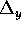)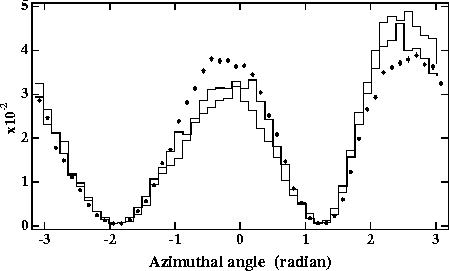Figure 9:   Azimuthal angular distributions measured for 6< r < 7cm at z=+1m for vertical displacements of=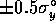(solid line),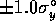(dotted line) compared with the nominal one (solid circles). The distributions are normalized by the total number of particles.

When two beams collide with a finite vertical displacement() comparable to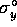, we expect a vertical, i.e.  up-down, asymmetry in the azimuthal angular distribution. In the simulation beam-1 and beam-2 are initially shifted by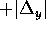and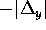, respectively. We examined the two cases of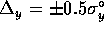and, and plotted the angular distribution in Fig.9. (The luminosity losses are 6 and 30% forand, respectively.) Apparently, during a collision the particles are deflected more in the upper side of the electromagnetic field of beam-2, since they are created in the overlapping region between two beams. Therefore, they are deflected more in +y and less in -y than in the nominal case, as can be clearly seen in Fig.9. The ratios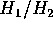are found to be 0.76 and 0.62 for=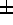0.5and1, respectively.

Toshiaki Tauchi
Sat Dec 21 00:34:16 JST 1996